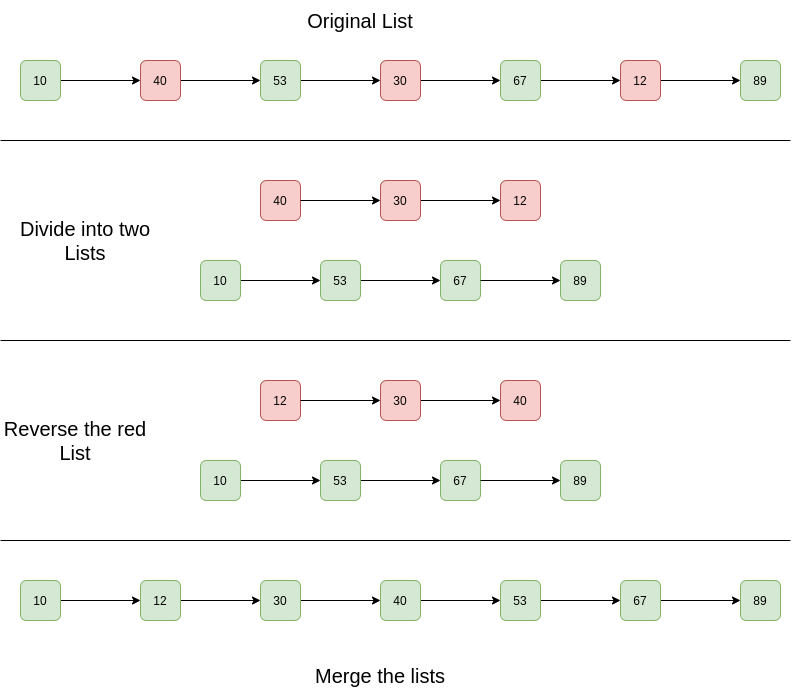# C++ Program For Sorting A Linked List That Is Sorted Alternating Ascending And Descending Orders

• Last Updated : 28 Dec, 2021

Given a Linked List. The Linked List is in alternating ascending and descending orders. Sort the list efficiently.

Example:

```Input List: 10 -> 40 -> 53 -> 30 -> 67 -> 12 -> 89 -> NULL
Output List: 10 -> 12 -> 30 -> 40 -> 53 -> 67 -> 89 -> NULL

Input List: 1 -> 4 -> 3 -> 2 -> 5 -> NULL
Output List: 1 -> 2 -> 3 -> 4 -> 5 -> NULL```

Simple Solution:
Approach: The basic idea is to apply to merge sort on the linked list.
Complexity Analysis:

• Time Complexity: The merge sort of linked list takes O(n log n) time. In the merge sort tree, the height is log n. Sorting each level will take O(n) time. So time complexity is O(n log n).
• Auxiliary Space: O(n log n), In the merge sort tree the height is log n. Storing each level will take O(n) space. So space complexity is O(n log n).

Efficient Solution:
Approach:

1. Separate two lists.
2. Reverse the one with descending order
3. Merge both lists.

Diagram:Below are the implementations of the above algorithm:

## C++

 `// C++ program to sort a linked``// list that is alternatively sorted ``// in increasing and decreasing order``#include ``using` `namespace` `std;`` ` `// Linked list node``struct` `Node ``{``    ``int` `data;``    ``struct` `Node* next;``};`` ` `Node* mergelist(Node* head1, ``                ``Node* head2);``void` `splitList(Node* head, ``               ``Node** Ahead, ``               ``Node** Dhead);``void` `reverselist(Node*& head);`` ` `// This is the main function that ``// sorts the linked list``void` `sort(Node** head)``{``    ``// Split the list into lists``    ``Node *Ahead, *Dhead;``    ``splitList(*head, &Ahead, &Dhead);`` ` `    ``// Reverse the descending linked list``    ``reverselist(Dhead);`` ` `    ``// Merge the two linked lists``    ``*head = mergelist(Ahead, Dhead);``}`` ` `// A utility function to create a ``// new node``Node* newNode(``int` `key)``{``    ``Node* temp = ``new` `Node;``    ``temp->data = key;``    ``temp->next = NULL;``    ``return` `temp;``}`` ` `// A utility function to reverse a ``// linked list``void` `reverselist(Node*& head)``{``    ``Node *prev = NULL, ``         ``*curr = head, *next;``    ``while` `(curr) ``    ``{``        ``next = curr->next;``        ``curr->next = prev;``        ``prev = curr;``        ``curr = next;``    ``}``    ``head = prev;``}`` ` `// A utility function to print ``// a linked list``void` `printlist(Node* head)``{``    ``while` `(head != NULL) ``    ``{``        ``cout << head->data << ``" "``;``        ``head = head->next;``    ``}``    ``cout << endl;``}`` ` `// A utility function to merge ``// two sorted linked lists``Node* mergelist(Node* head1, ``                ``Node* head2)``{``    ``// Base cases``    ``if` `(!head1)``        ``return` `head2;``    ``if` `(!head2)``        ``return` `head1;`` ` `    ``Node* temp = NULL;``    ``if` `(head1->data < head2->data) ``    ``{``        ``temp = head1;``        ``head1->next = mergelist(head1->next, ``                                ``head2);``    ``}``    ``else` `    ``{``        ``temp = head2;``        ``head2->next = mergelist(head1, ``                                ``head2->next);``    ``}``    ``return` `temp;``}`` ` `// This function alternatively splits``// a linked list with head as head into two:``// For example, 10->20->30->15->40->7 ``// is splitted into 10->30->40 and 20->15->7``// "Ahead" is reference to head of ascending ``// linked list``// "Dhead" is reference to head of descending ``// linked list``void` `splitList(Node* head, Node** Ahead, ``               ``Node** Dhead)``{``    ``// Create two dummy nodes to ``    ``// initialize heads of two ``    ``// linked list``    ``*Ahead = newNode(0);``    ``*Dhead = newNode(0);`` ` `    ``Node* ascn = *Ahead;``    ``Node* dscn = *Dhead;``    ``Node* curr = head;`` ` `    ``// Link alternate nodes``    ``while` `(curr) ``    ``{``        ``// Link alternate nodes of ascending ``        ``// linked list``        ``ascn->next = curr;``        ``ascn = ascn->next;``        ``curr = curr->next;`` ` `        ``// Link alternate nodes of descending ``        ``// linked list``        ``if` `(curr) ``        ``{``            ``dscn->next = curr;``            ``dscn = dscn->next;``            ``curr = curr->next;``        ``}``    ``}`` ` `    ``ascn->next = NULL;``    ``dscn->next = NULL;``    ``*Ahead = (*Ahead)->next;``    ``*Dhead = (*Dhead)->next;``}`` ` `// Driver code``int` `main()``{``    ``Node* head = newNode(10);``    ``head->next = newNode(40);``    ``head->next->next = newNode(53);``    ``head->next->next->next = ``    ``newNode(30);``    ``head->next->next->next->next = ``    ``newNode(67);``    ``head->next->next->next->next->next = ``    ``newNode(12);``    ``head->next->next->next->next->next->next = ``    ``newNode(89);`` ` `    ``cout << ``"Given Linked List is "` `<< endl;``    ``printlist(head);`` ` `    ``sort(&head);`` ` `    ``cout << ``"Sorted Linked List is "` `<< endl;``    ``printlist(head);`` ` `    ``return` `0;``}`

Output:

```Given Linked List is
10 40 53 30 67 12 89
10 12 30 40 53 67 89```

Complexity Analysis:

• Time Complexity: O(n).
One traversal is needed to separate the list and reverse them. The merging of sorted lists takes O(n) time.
• Auxiliary Space: O(1).
No extra space is required.

Please refer complete article on Sort a linked list that is sorted alternating ascending and descending orders? for more details!

My Personal Notes arrow_drop_up